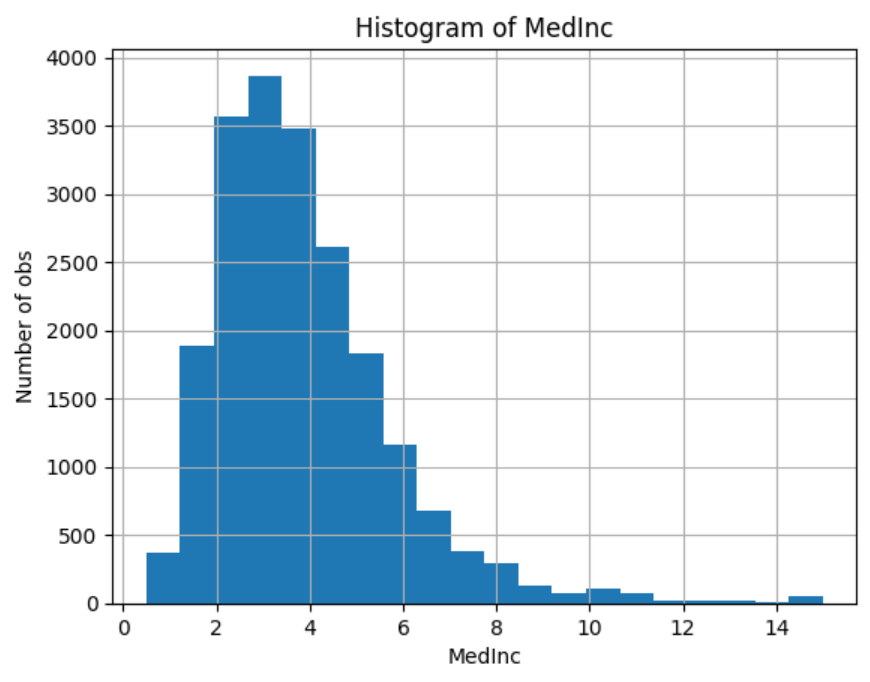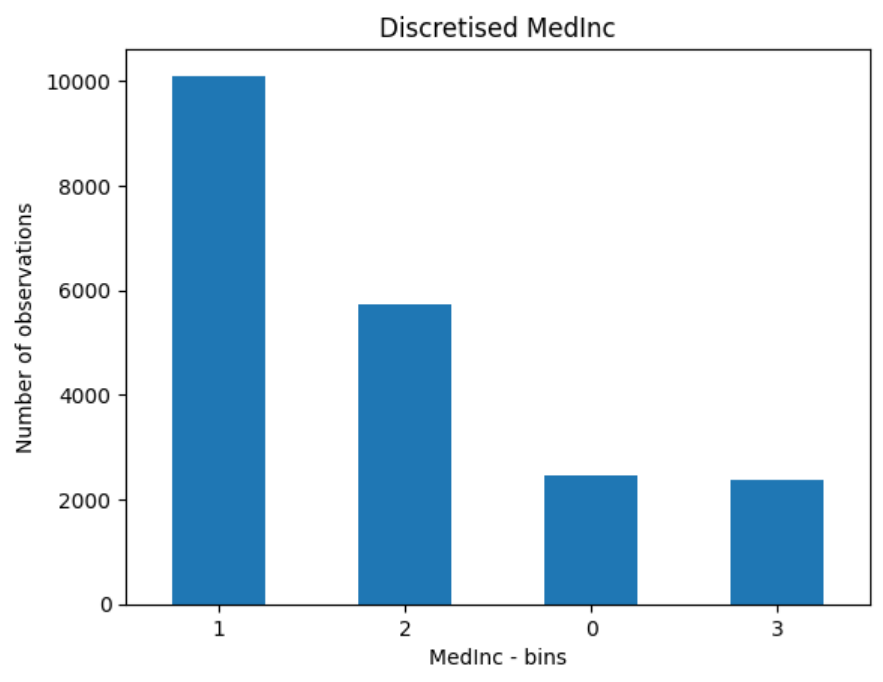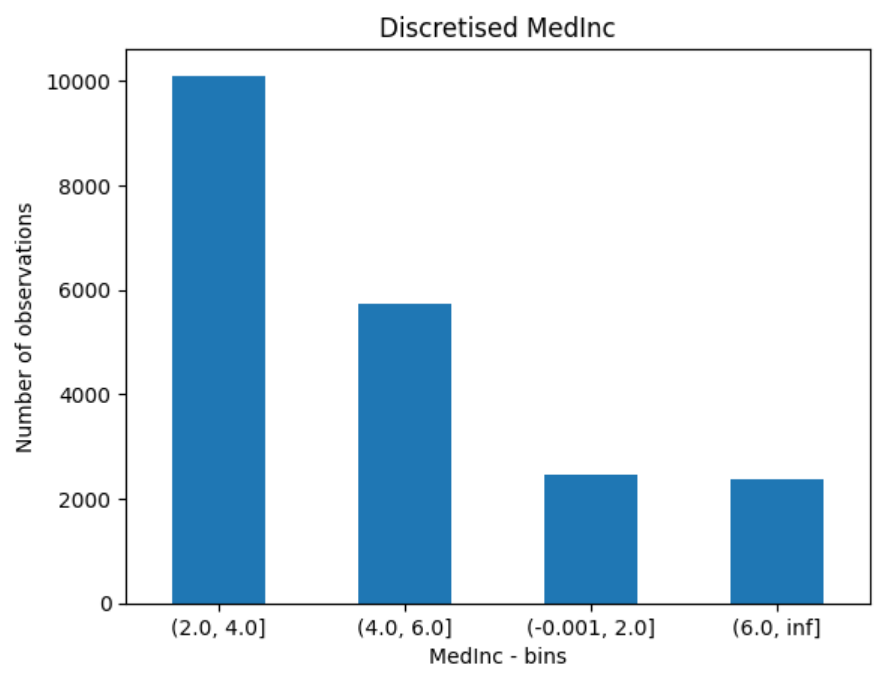# ArbitraryDiscretiser#

The `ArbitraryDiscretiser()` sorts the variable values into contiguous intervals which limits are arbitrarily defined by the user. Thus, you must provide a dictionary with the variable names as keys and a list with the limits of the intervals as values, when setting up the discretiser.

The `ArbitraryDiscretiser()` works only with numerical variables. The discretiser will check that the variables entered by the user are present in the train set and cast as numerical.

## Example#

Let’s take a look at how this transformer works. First, let’s load a dataset and plot a histogram of a continuous variable. We use the california housing dataset that comes with Scikit-learn.

```import numpy as np
import pandas as pd
import matplotlib.pyplot as plt
from sklearn.datasets import fetch_california_housing
from feature_engine.discretisation import ArbitraryDiscretiser

X, y = fetch_california_housing( return_X_y=True, as_frame=True)

X['MedInc'].hist(bins=20)
plt.xlabel('MedInc')
plt.ylabel('Number of obs')
plt.title('Histogram of MedInc')
plt.show()
```

In the following plot we see a histogram of the variable median income:Now, let’s discretise the variable into arbitrarily determined intervals. We want the intervals as integers in the resulting transformation, so we set `return_boundaries` to `False`.

```user_dict = {'MedInc': [0, 2, 4, 6, np.Inf]}

transformer = ArbitraryDiscretiser(
binning_dict=user_dict, return_object=False, return_boundaries=False)

X = transformer.fit_transform(X)
```

Now, we can go ahead and plot the variable after the transformation:

```X['MedInc'].value_counts().plot.bar(rot=0)
plt.xlabel('MedInc - bins')
plt.ylabel('Number of observations')
plt.title('Discretised MedInc')
plt.show()
```

In the following plot we see the number of observations per interval:Note that in the above figure the intervals are represented by digits.

Alternatively, we can return the interval limits in the discretised variable by setting `return_boundaries` to `True`.

```X, y = fetch_california_housing( return_X_y=True, as_frame=True)

user_dict = {'MedInc': [0, 2, 4, 6, np.Inf]}

transformer = ArbitraryDiscretiser(
binning_dict=user_dict, return_object=False, return_boundaries=True)
X = transformer.fit_transform(X)

X['MedInc'].value_counts().plot.bar(rot=0)
plt.xlabel('MedInc - bins')
plt.ylabel('Number of observations')
plt.title('Discretised MedInc')
plt.show()
```

In the following plot we see the number of observations per interval:Discretisation plus encoding

If we return the interval values as integers, the discretiser has the option to return the transformed variable as integer or as object. Why would we want the transformed variables as object?

Categorical encoders in Feature-engine are designed to work with variables of type object by default. Thus, if you wish to encode the returned bins further, say to try and obtain monotonic relationships between the variable and the target, you can do so seamlessly by setting `return_object` to True. You can find an example of how to use this functionality here.

Check also: